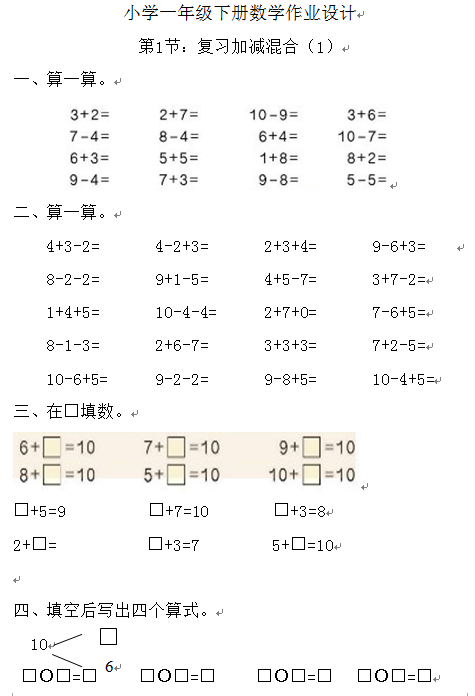ceomax主题已启用，当前站点还没有验证正版主题授权，暂不可使用 前往授权激活 获取正版授权
【DOC文档71页】小学新课标人教版一年级下册数学练习题全套 A4电子版资料_可直接打印_会员免费下载 - 悟思学_领悟_思考_学习

【DOC文档71页】小学新课标人教版一年级下册数学练习题全套 A4电子版资料_可直接打印_会员免费下载

317

1. ：复习加减混合
2. 算一算。

4+3-2=       4-2+3=       2+3+4=       9-6+3=

8-2-2=       9+1-5=       4+5-7=       3+7-2=

1+4+5=       10-4-4=      2+7+0=       7-6+5=

【DOC文档71页】小学新课标人教版一年级下册数学练习题全套 A4电子版资料_可直接打印_会员免费下载相关资料253 5687 2457 2458 2

• 0 +

访问总数

• 0 +

会员总数

• 0 +

文档总数

• 0 +

今日发布

• 0 +

本周发布

• 0 +

运行天数